Do you like the article?
Share it with others -
Use new possibilities of MetaTrader 5

# MQL5 Cookbook: Development of a Multi-Symbol Indicator to Analyze Price Divergence

29 April 2014, 17:34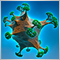0
10 005

### Introduction

In this article, we will consider the development of a multi-symbol indicator to analyze price divergence in a specified period of time. The core topics have been already discussed in the previous article on the programming of multi-currency indicators MQL5 Cookbook: Developing a Multi-Symbol Volatility Indicator in MQL5. So this time we will dwell only on those new features and functions that have been changed dramatically. If you are new to the programming of multi-currency indicators, I recommend you to first read the previous article.

• Changing the chart properties.
• Handling of the CHARTEVENT_OBJECT_DRAG (dragging a chart object) and CHARTEVENT_CHART_CHANGE (resizing the chart or modifying the chart properties using the properties dialog window) events.
• Rendering indicator buffers using more than one color.
• Defining highs and lows in indicator buffers within the visibility area to set a chart high/low.
• Inversion of a series.

The resulting amount of code for our indicator is quite large, about 1500 lines. Therefore, we will distribute all the functions in separate files and will link them to the main project file. There will be three categories of functions for external files:

• Checks.mqh - Functions to perform various checks and to download available data.
• Objects.mqh - Functions for managing graphical objects.
• Chart.mqh - Functions for managing chart properties.

All the functions that do not belong to the categories above will be left in the main file.

### Development of the Indicator

Next proceed to the programming of indicator. First we need to create a new project. To do this, in the Metatrader 5\MQL5\Indicators directory create folder named as our indicator, and in it the Include folder to which we will place the include files. Next, create the main file in the indicator folder. This can be done manually by creating a text file with the *.mq5 extension or using the MQL5 Wizard by template. In addition to the core functions of the program OnInit(), OnDeinit() and OnCalculate(), we will also use OnChartEvent() and OnTimer().

Just like in the previous article, in addition to the current symbol we will display data for 5 symbols specified in external parameters. But this time, instead of values calculated by some formula, we will output raw price data on the chart. User is free to choose the type of data representation in the external parameters from the drop-down list: Line, Bars or Candlesticks.

If we would only have to display data as one-colored lines, then it will be enough to specify the number of buffers equal to the number of symbols in the indicator properties (#property). But since there are two modes for drawing series as bars and candles, we need more buffers for the two-color mode: four buffers to render each series and one buffer to set the color (depending on condition) for each element in a graphic series.

For each series it is required to specify colors in the program properties section. To do this, simply list them separated by commas. First comes the color used in one-color mode. In two-color mode, it is used for the up bars/candlesticks. The second color will be used only in the two-color mode for the down bars/candlesticks.

The codes of all these parameters are provided below:

```#property indicator_chart_window // Indicator is in the main window
#property indicator_buffers 25   // Number of buffers for indicator calculation
#property indicator_plots   5    // Number of plotting series
//--- Indicator buffers colors
#property indicator_color1  clrDodgerBlue,C'0,50,100'
#property indicator_color2  clrLimeGreen,C'20,80,20'
#property indicator_color3  clrGold,C'160,140,0'
#property indicator_color4  clrAqua,C'0,140,140'
#property indicator_color5  clrMagenta,C'130,0,130'
```

With the #define directive let's declare constants and using the #include command line let's include files with functions that have already been described above, and the class from Standard library to work with the canvas:

```//--- Constants
#define RESET           0 // Returning the indicator recalculation command to the terminal
#define SYMBOLS_COUNT   5 // Number of symbols
//--- Include the class for working with the canvas
#include <Canvas\Canvas.mqh>
//--- Include the class for working with the canvas
#include "Include/Checks.mqh"
#include "Include/Chart.mqh"
#include "Include/Objects.mqh"

```

Add the ENUM_DRAWTYPE and ENUM_START_POINT enumerations to create drop-down lists that allow to select drawing type of the price data and mode of the price divergence starting point in external parameters:

```//--- Drawing type of the price data
enum ENUM_DRAWTYPE
{
LINE   =0,  // Line
BARS   =1,  // Bars
CANDLES=2   // Candlesticks
};
//--- Mode of the price divergence starting point
enum ENUM_START_POINT
{
VERTICAL_LINE=0,  // Vertical line
MONTH        =1,  // Month
WEEK         =2,  // Week
DAY          =3,  // Day
HOUR         =4   // Hour
};
```

The types of data rendering is already described above, now let's talk a bit more about what the price divergence starting point means.

Altogether there will be five modes: Vertical line, Month, Week, Day and Hour. For the Vertical line mode, a vertical line will be added when loading indicator on the chart. By dragging this line you specify the bar where prices of all symbols will meet in a single point. The open price of specified bar for the current symbol will be considered as a reference point of this meeting. Any other mode will tell the program that each time prices should meet at the beginning of specified period. I.e. at the beginning of each month, at the beginning of each week, at the beginning of each day or at the beginning of each hour.

Below you can find the list of the input parameters of the indicator:

```//--- External parameters
input  ENUM_DRAWTYPE    DrawType             =CANDLES;       // Drawing type
input  ENUM_START_POINT StartPriceDivergence =VERTICAL_LINE; // Start of price divergence
input  bool             TwoColor             =false;         // Two-color bars/candlesticks
sinput string dlm01=""; //- - - - - - - - - - - - - - - - - - - - - - - - - - - - - - - - - - - -
input  string           Symbol02             ="GBPUSD";      // Symbol 2
input  bool             Inverse02            =false;         // Inverse symbol 2
input  string           Symbol03             ="AUDUSD";      // Symbol 3
input  bool             Inverse03            =false;         // Inverse symbol 3
input  string           Symbol04             ="NZDUSD";      // Symbol 4
input  bool             Inverse04            =false;         // Inverse symbol 4
input  string           Symbol05             ="USDCAD";      // Symbol 5
input  bool             Inverse05            =false;         // Inverse symbol 5
input  string           Symbol06             ="USDCHF";      // Symbol 6
input  bool             Inverse06            =false;         // Inverse symbol 6
```

Symbols are numbered starting from 2, since 1 is the current symbol on chart.

Inversion can be applied for each included symbol. Inversion means that the symbol data will be rendered upside down. This can be useful, when the list of the symbols analyzed includes currency pairs in which the same currency (for example, US dollar) can be both the base one and the counter one. For example, in the EURUSD currency pair, US dollar is the counter currency, and in the USDCHF currency pair it is the base one. If the current symbol on the chart is EURUSD, then you can turn on the inversion for USDCHF, what will make the representation of the prices more convenient for the analysis.

Below is the list of the global variables and arrays:

```//--- Structure of the indicator buffers arrays
struct buffers
{
double            open[];   // Open prices buffer
double            high[];   // High prices buffer
double            low[];    // Low prices buffer
double            close[];  // Close prices buffer
double            icolor[]; // Buffer to determine the color of element
};
buffers           buffer_data[SYMBOLS_COUNT];
CCanvas           canvas;
//--- Variables/arrays for copying data from OnCalculate()
int               OC_rates_total     =0; // Size of input time series
int               OC_prev_calculated =0; // Bars processed at the previous call
datetime          OC_time[];             // Opening time
double            OC_open[];             // Open prices
double            OC_high[];             // High prices
double            OC_low[];              // Low prices
double            OC_close[];            // Close prices
long              OC_tick_volume[];      // Tick volumes
long              OC_volume[];           // Real volumes

//--- For the purpose of storing and checking the time of the first bar in the terminal
datetime          series_first_date[SYMBOLS_COUNT];
datetime          series_first_date_last[SYMBOLS_COUNT];
//--- Time array of the bar from which we will start drawing
datetime          limit_time[SYMBOLS_COUNT];
//--- Symbol names array
string            symbol_names[SYMBOLS_COUNT];
//--- Array of symbol inverse flags
bool              inverse[SYMBOLS_COUNT];
//--- Colors of indicator lines
color             line_colors[SYMBOLS_COUNT]={clrDodgerBlue,clrLimeGreen,clrGold,clrAqua,clrMagenta};
//--- String representing the lack of the symbol
string            empty_symbol="EMPTY";
//--- Chart properties
int               window_number              =WRONG_VALUE;               // Indicator window number
int               chart_width                =0;                         // Chart width
int               chart_height               =0;                         // Chart height
int               last_chart_width           =0;                         // Last saved chart width
int               last_chart_height          =0;                         // Last saved chart height
int               chart_center_x             =0;                         // Horizontal center of chart
int               chart_center_y             =0;                         // Vertical center of chart
color             color_bar_up               =clrRed;                    // Up bar color
color             color_bar_down             =C'100,0,0';                // Down bar color
string            indicator_shortname        ="MS_PriceDivergence";      // Short name of the indicator
string            prefix                     =indicator_shortname+"_";   // Prefix for objects
//--- Name of vertical line of the price divergence starting point
string            start_price_divergence=prefix+"start_price_divergence";
//--- Canvas properties
string            canvas_name             =prefix+"canvas";          // Canvas name
color             canvas_background       =clrBlack;                 // Canvas background color
uchar             canvas_opacity          =190;                      // Opacity
int               font_size               =16;                       // Font size
string            font_name               ="Calibri";                // Font
ENUM_COLOR_FORMAT clr_format              =COLOR_FORMAT_ARGB_RAW;    // Color components should be correctly set by the user
//--- Canvas messages
string            msg_prepare_data        ="Preparing data! Please wait...";
string            msg_not_synchronized    ="Unsynchronized data! Please wait...";
string            msg_sync_update         ="";
string            msg_last                ="";
//---
ENUM_TIMEFRAMES   timeframe_start_point  =Period();    // Timeframe for the price divergence starting point
datetime          first_period_time      =NULL;        // Time of the first specified period on chart
double            divergence_price       =0.0;         // Price of the price divergence starting point
datetime          divergence_time        =NULL;        // Time of the price divergence starting point
double            symbol_difference[SYMBOLS_COUNT];    // Difference in price relative to the current symbol
double            inverse_difference[SYMBOLS_COUNT];   // Difference that is formed when calculating inversion
```

Next, we will consider functions that are used during the indicator initialization. In general, there are no major changes compared with the OnInit() function from the previous article.

Let's add the check on where the indicator is used. The point is that currently the terminal developers have not implemented all the features of controlling the chart properties in Strategy Tester, so we restrict our indicator to be used only out of Strategy Tester. To implement this, we will write a simple function - CheckTesterMode(). It will be located in the Checks.mqh file:

```//+------------------------------------------------------------------+
//| Checks if indicator is used in Strategy Tester                   |
//+------------------------------------------------------------------+
bool CheckTesterMode()
{
//--- Report that indicator is not intended to be used in Strategy Tester
if(MQLInfoInteger(MQL_TESTER) ||
MQLInfoInteger(MQL_VISUAL_MODE) ||
MQLInfoInteger(MQL_OPTIMIZATION))
{
Comment("Currently, the <- "+MQLInfoString(MQL_PROGRAM_NAME)+" -> indicator is not intended to be used in Strategy Tester!");
return(false);
}
//---
return(true);
}
```

Another new function SetBarsColors() is intended to set colors for bars/candlesticks of current symbol. It is located in the Chart.mqh file.

```//+------------------------------------------------------------------+
//| Sets colors for the current symbol bars                          |
//+------------------------------------------------------------------+
void SetBarsColors()
{
//--- Color for the up bar, shadows and body borders of bull candlesticks
ChartSetInteger(0,CHART_COLOR_CHART_UP,color_bar_up);
//--- Body color of a bull candlestick
ChartSetInteger(0,CHART_COLOR_CANDLE_BULL,color_bar_up);
//--- Line chart color and color of "Doji" Japanese candlesticks
ChartSetInteger(0,CHART_COLOR_CHART_LINE,color_bar_up);
//--- For two-color mode
if(TwoColor)
{
//--- Color for the down bar, shadows and body borders of bear candlesticks
ChartSetInteger(0,CHART_COLOR_CHART_DOWN,color_bar_down);
//--- Body color of a bear candlestick
ChartSetInteger(0,CHART_COLOR_CANDLE_BEAR,color_bar_down);
}
//--- If two-color mode is turned off
else
{
//--- Color for the down bar, shadows and body borders of bear candlesticks
ChartSetInteger(0,CHART_COLOR_CHART_DOWN,color_bar_up);
//--- Body color of a bear candlestick
ChartSetInteger(0,CHART_COLOR_CANDLE_BEAR,color_bar_up);
}
}
```

During the initialization, we need to determine which mode is selected in the StartPriceDivergence external parameter. If the Vertical line is selected, then the timeframe_start_point global variable will be assigned with default value, i.e. current timeframe. Otherwise selected timeframe will be applied. For this purpose, let's write the InitStartPointTF() function:

```//+------------------------------------------------------------------+
//| Identifies timeframe for the price starting point mode           |
//+------------------------------------------------------------------+
void InitStartPointTF()
{
//--- Exit if vertical line mode is selected
if(StartPriceDivergence==VERTICAL_LINE)
return;
//--- Otherwise define the timeframe
switch(StartPriceDivergence)
{
case MONTH : timeframe_start_point=PERIOD_MN1; break;
case WEEK  : timeframe_start_point=PERIOD_W1;  break;
case DAY   : timeframe_start_point=PERIOD_D1;  break;
case HOUR  : timeframe_start_point=PERIOD_H1;  break;
}
}
```

The CheckInputParameters() function unlike the one from the previous article now looks like this:

```//+------------------------------------------------------------------+
//| Checks input parameters for correctness                          |
//+------------------------------------------------------------------+
bool CheckInputParameters()
{
//--- For all other modes except the 'Vertical Line'
if(StartPriceDivergence!=VERTICAL_LINE)
{
//--- If the current period is greater than or equal to the specified period of the price divergence starting point, report of it and exit
if(PeriodSeconds()>=PeriodSeconds(timeframe_start_point))
{
Print("Current timeframe should be less than one specified in the Start Price Divergence parameter!");
Comment("Current timeframe should be less than one specified in the Start Price Divergence parameter!");
return(false);
}
}
//---
return(true);
}
```

Arrays are initialized just like in the previous article. Only the names and the number of arrays have been changed.

```//+------------------------------------------------------------------+
//| First initialization of arrays                                   |
//+------------------------------------------------------------------+
void InitArrays()
{
ArrayInitialize(limit_time,NULL);
ArrayInitialize(symbol_difference,0.0);
ArrayInitialize(inverse_difference,0.0);
ArrayInitialize(series_first_date,NULL);
ArrayInitialize(series_first_date_last,NULL);
//---
for(int s=0; s<SYMBOLS_COUNT; s++)
{
ArrayInitialize(buffer_data[s].open,EMPTY_VALUE);
ArrayInitialize(buffer_data[s].high,EMPTY_VALUE);
ArrayInitialize(buffer_data[s].low,EMPTY_VALUE);
ArrayInitialize(buffer_data[s].close,EMPTY_VALUE);
ArrayInitialize(buffer_data[s].icolor,EMPTY_VALUE);
}
}
//+------------------------------------------------------------------+
//| Initializes array of symbols                                     |
//+------------------------------------------------------------------+
void InitSymbolNames()
{
}
//+------------------------------------------------------------------+
//| Initializes array of inversions                                  |
//+------------------------------------------------------------------+
void InitInverse()
{
inverse=Inverse02;
inverse=Inverse03;
inverse=Inverse04;
inverse=Inverse05;
inverse=Inverse06;
}
```

Significant changes were made to the SetIndicatorProperties() function. In fact, this is completely new function. Now, depending on which mode of data rendering is selected, the corresponding properties are set during initialization.

```//+------------------------------------------------------------------+
//| Sets indicator properties                                        |
//+------------------------------------------------------------------+
void SetIndicatorProperties()
{
//--- Set the short name
IndicatorSetString(INDICATOR_SHORTNAME,indicator_shortname);
//--- Set the number of decimal digits
IndicatorSetInteger(INDICATOR_DIGITS,_Digits);
//---  In the 'Line' mode we need only one buffers that displays the open price
if(DrawType==LINE)
{
for(int s=0; s<SYMBOLS_COUNT; s++)
SetIndexBuffer(s,buffer_data[s].close,INDICATOR_DATA);
}
//--- In other modes we use all prices for drawing
//    bars/candlesticks and additional buffer for the two-color mode
else if(DrawType==BARS || DrawType==CANDLES)
{
for(int s=0; s<SYMBOLS_COUNT; s++)
{
static int buffer_number=0;
SetIndexBuffer(buffer_number,buffer_data[s].open,INDICATOR_DATA);
buffer_number++;
SetIndexBuffer(buffer_number,buffer_data[s].high,INDICATOR_DATA);
buffer_number++;
SetIndexBuffer(buffer_number,buffer_data[s].low,INDICATOR_DATA);
buffer_number++;
SetIndexBuffer(buffer_number,buffer_data[s].close,INDICATOR_DATA);
buffer_number++;
SetIndexBuffer(buffer_number,buffer_data[s].icolor,INDICATOR_COLOR_INDEX);
buffer_number++;
}
}
//--- Set labels for the current timeframe
//    In the 'Line' mode only opening price is used
if(DrawType==LINE)
{
for(int s=0; s<SYMBOLS_COUNT; s++)
PlotIndexSetString(s,PLOT_LABEL,symbol_names[s]+",Close");
}
//--- In other modes all prices of bars/candlesticks
//    ";" is used as a separator
else if(DrawType==BARS || DrawType==CANDLES)
{
for(int s=0; s<SYMBOLS_COUNT; s++)
{
PlotIndexSetString(s,PLOT_LABEL,
symbol_names[s]+",Open;"+
symbol_names[s]+",High;"+
symbol_names[s]+",Low;"+
symbol_names[s]+",Close");
}
}
//--- Set the type of lines for indicator buffers
//--- Line
if(DrawType==LINE)
for(int s=0; s<SYMBOLS_COUNT; s++)
PlotIndexSetInteger(s,PLOT_DRAW_TYPE,DRAW_LINE);
//--- Bars
if(DrawType==BARS)
for(int s=0; s<SYMBOLS_COUNT; s++)
PlotIndexSetInteger(s,PLOT_DRAW_TYPE,DRAW_COLOR_BARS);
//--- Candlesticks
if(DrawType==CANDLES)
for(int s=0; s<SYMBOLS_COUNT; s++)
PlotIndexSetInteger(s,PLOT_DRAW_TYPE,DRAW_COLOR_CANDLES);

//--- Set the type of lines for data of current symbol
//--- Line
if(DrawType==LINE)
ChartSetInteger(0,CHART_MODE,CHART_LINE);
//--- Bars
if(DrawType==BARS)
ChartSetInteger(0,CHART_MODE,CHART_BARS);
//--- Candlesticks
if(DrawType==CANDLES)
ChartSetInteger(0,CHART_MODE,CHART_CANDLES);

//--- Set the line width
for(int s=0; s<SYMBOLS_COUNT; s++)
PlotIndexSetInteger(s,PLOT_LINE_WIDTH,1);
//--- Set the line color for the 'Line' mode
if(DrawType==LINE)
for(int s=0; s<SYMBOLS_COUNT; s++)
PlotIndexSetInteger(s,PLOT_LINE_COLOR,line_colors[s]);
//--- Display data in Data Window only for existing symbols
for(int s=0; s<SYMBOLS_COUNT; s++)
{
if(symbol_names[s]!=empty_symbol)
PlotIndexSetInteger(s,PLOT_SHOW_DATA,true);
else
PlotIndexSetInteger(s,PLOT_SHOW_DATA,false);
}
//--- Empty value for plotting where nothing will be drawn
for(int s=0; s<SYMBOLS_COUNT; s++)
PlotIndexSetDouble(s,PLOT_EMPTY_VALUE,EMPTY_VALUE);
}
```

And finally, another new function SetDivergenceLine() to be used in OnInit(). It sets the vertical green line to manipulate the price divergence start point in the Vertical line mode.

```//+------------------------------------------------------------------+
//| Sets vertical line for price divergence starting point           |
//+------------------------------------------------------------------+
void SetDivergenceLine()
{
//--- If there is no vertical line yet, set it
if(StartPriceDivergence==VERTICAL_LINE && ObjectFind(0,start_price_divergence)<0)
//--- Place a vertical line on the true bar
CreateVerticalLine(0,0,TimeCurrent()+PeriodSeconds(),start_price_divergence,
2,STYLE_SOLID,clrGreenYellow,true,true,false,"","\n");
//--- For all other modes except the 'Vertical Line'
if(StartPriceDivergence!=VERTICAL_LINE)
DeleteObjectByName(start_price_divergence);
}
```

Below is the representation of everything described above inside the OnInit() function. When everything is split into separate functions and files, it becomes very convenient to read the program code.

```//+------------------------------------------------------------------+
//| Custom indicator initialization function                         |
//+------------------------------------------------------------------+
int OnInit()
{
//--- Check if indicator is currently being used in Strategy Tester
if(!CheckTesterMode())
return(INIT_FAILED);
//--- Set the color for bars/candlesticks
SetBarsColors();
//--- Define the timeframe for the price divergence starting point
InitStartPointTF();
//--- Check input parameters for correctness
if(!CheckInputParameters())
return(INIT_PARAMETERS_INCORRECT);
//--- Set the timer at 1-second intervals
EventSetMillisecondTimer(1000);
//--- Set the font to be displayed on the canvas
canvas.FontSet(font_name,font_size,FW_NORMAL);
//--- Initialization of arrays
InitArrays();
//--- Initialize the array of symbols
InitSymbolNames();
//--- Initialize the array of inversions
InitInverse();
//--- Set indicator properties
SetIndicatorProperties();
//--- Set vertical line of the price divergence start
SetDivergenceLine();
//--- Clear the comment
Comment("");
//--- Refresh the chart
ChartRedraw();
//--- Initialization completed successfully
return(INIT_SUCCEEDED);
}
```

In the OnCalculate() function, the program code remained virtually unchanged. In the previous article, after all the checks on the availability of data have been made, the program first filled auxiliary arrays and only then filled indicator buffers with prepared data. This time we will try to arrange everything in a single loop.

I made functions of validating and loading data more strict. Now each value you want to get passes through specified number of attempts. If value is obtained, the loop is stopped. And since now we have modes where we need to determine the start of a period (month, week, day, hour), then we will get the period start time via the higher timeframe. Therefore, I created an additional function similar to LoadAndFormData() which has a similar name of LoadAndFormDataHighTF(). Its code is very similar to the original one, so I will not post it here.

Verification of the data availability for the current and higher timeframes was implemented in one function CheckAvailableData():

```//+------------------------------------------------------------------+
//| Checks the amount of available data for all symbols              |
//+------------------------------------------------------------------+
bool CheckAvailableData()
{
int attempts=100;

//---
for(int s=0; s<SYMBOLS_COUNT; s++)
{
//--- If this symbol is available
if(symbol_names[s]!=empty_symbol)
{
datetime time[];                    // Array for checking the number of bars
int      total_period_bars   =0;    // Number of bars of the current period
datetime terminal_first_date =NULL; // First date of the current time frame data available in the terminal
//--- Get the first date of the current time frame data in the terminal
terminal_first_date=(datetime)SeriesInfoInteger(symbol_names[s],Period(),SERIES_TERMINAL_FIRSTDATE);
//--- Get the number of available bars from the date specified
total_period_bars=Bars(symbol_names[s],Period(),terminal_first_date,TimeCurrent());
//--- Check the readiness of bar data
for(int i=0; i<attempts; i++)
{
//--- Copy the specified amount of data
if(CopyTime(symbol_names[s],Period(),0,total_period_bars,time))
{
//--- If the required amount has been copied, terminate the loop
if(ArraySize(time)>=total_period_bars)
break;
}
}
//--- If the amount of data copied is not sufficient, one more attempt is required
if(ArraySize(time)==0 || ArraySize(time)<total_period_bars)
{
msg_last=msg_prepare_data;
ShowCanvasMessage(msg_prepare_data);
OC_prev_calculated=0;
return(false);
}
}
}
//--- Exit if current mode is vertical line of the price divergence starting point
if(StartPriceDivergence==VERTICAL_LINE)
return(true);
else
{
datetime time[];                    // Array for checking the number of bars
int      total_period_bars   =0;    // Number of bars of the current period
datetime terminal_first_date =NULL; // First date of the current time frame data available in the terminal
//--- Get the first date of the current time frame data in the terminal
for(int i=0; i<attempts; i++)
if((terminal_first_date=(datetime)SeriesInfoInteger(Symbol(),Period(),SERIES_FIRSTDATE))>0)
break;
//--- Get the number of available bars from the date specified
for(int i=0; i<attempts; i++)
if((total_period_bars=(int)SeriesInfoInteger(Symbol(),timeframe_start_point,SERIES_BARS_COUNT))>0)
break;
//--- Check the readiness of bar data
for(int i=0; i<attempts; i++)
//--- Copy the specified amount of data
if(CopyTime(Symbol(),timeframe_start_point,
terminal_first_date+PeriodSeconds(timeframe_start_point),TimeCurrent(),time)>0)
break;
//--- If the amount of data copied is not sufficient, one more attempt is required
if(ArraySize(time)<=0 || total_period_bars<=0)
{
msg_last=msg_prepare_data;
ShowCanvasMessage(msg_prepare_data);
OC_prev_calculated=0;
return(false);
}
}
//---
return(true);
}
```

The FillIndicatorBuffers() function has been significantly complicated for the current task. This is due to the fact that now there are several modes, and each one of them requires its own actions. In fact, everything can be divided into four steps.

• Getting data for specified symbol.
• Getting data for higher timeframe, and determining the time and the price level, where the prices of all the symbols are meeting.
• Calculating values and filling the indicator buffer.
• Verification of the calculated values.

```//+------------------------------------------------------------------+
//| Fills indicator buffers                                          |
//+------------------------------------------------------------------+
void FillIndicatorBuffers(int i,int s,datetime const &time[])
{
MqlRates    rates[];             // Data structure
double      period_open[];       // Opening price for bar at the price divergence starting point
datetime    period_time[];       // Time of the price divergence starting point
int         attempts=100;        // Number of copying attempts
datetime    high_tf_time=NULL;   // Time of higher timeframe's bar

//--- Exit if we are out of "true" bars zone
if(time[i]<limit_time[s])
return;
//--- Reset the last error
ResetLastError();
//--- Get data of current bar for the specified symbol
for(int j=0; j<attempts; j++)
if(CopyRates(symbol_names[s],Period(),time[i],1,rates)==1)
{ ResetLastError(); break; }
//--- Exit if failed to get data
if(ArraySize(rates)<1 || GetLastError()!=0)
return;
//--- If the current time is before the first timeframe's time or
//    bar time is not equal to the bar time of the current symbol or
//    empty values are fetched
if(rates.time==NULL ||
time[i]!=rates.time ||
time[i]<first_period_time ||
rates.low==EMPTY_VALUE ||
rates.open==EMPTY_VALUE ||
rates.high==EMPTY_VALUE ||
rates.close==EMPTY_VALUE)
{
//--- Write empty value
if(DrawType!=LINE)
{
buffer_data[s].low[i]   =EMPTY_VALUE;
buffer_data[s].open[i]  =EMPTY_VALUE;
buffer_data[s].high[i]  =EMPTY_VALUE;
}
buffer_data[s].close[i]=EMPTY_VALUE;
return;
}
//--- If current mode is vertical line of the price divergence starting point
if(StartPriceDivergence==VERTICAL_LINE)
{
//--- Get the time of the line
divergence_time=(datetime)ObjectGetInteger(0,start_price_divergence,OBJPROP_TIME);
//--- Get the time of the first bar
first_period_time=time;
}
//--- For all other modes, we will keep track the beginning of period
else
{
//--- If we are here for the first time, store data of the first bar of higher timeframe
if(divergence_time==NULL)
{
ResetLastError();
//--- Get opening time of the first bar of higher timeframe
for(int j=0; j<attempts; j++)
if(CopyTime(Symbol(),timeframe_start_point,time+PeriodSeconds(timeframe_start_point),1,period_time)==1)
{ ResetLastError(); break; }
//--- Exit if failed to get price/time
if(ArraySize(period_time)<1 || GetLastError()!=0)
return;
//--- Otherwise store time of the first bar of higher timeframe
else
first_period_time=period_time;
}
//--- If current bar's time on the current timeframe is before the first bar's time on higher timeframe
if(time[i]<first_period_time)
high_tf_time=first_period_time;
//--- Otherwise we will receive data of the last bar of the higher timeframe with respect to the current bar on the current timeframe
else
high_tf_time=time[i];
//--- Reset the last error
ResetLastError();
//--- Get the opening price of the first bar of the higher timeframe
for(int j=0; j<attempts; j++)
if(CopyOpen(Symbol(),timeframe_start_point,high_tf_time,1,period_open)==1)
{ ResetLastError(); break; }
//--- Get opening time of the first bar of higher timeframe
for(int j=0; j<attempts; j++)
if(CopyTime(Symbol(),timeframe_start_point,high_tf_time,1,period_time)==1)
{ ResetLastError(); break; }
//--- Exit if failed to get price/time
if(ArraySize(period_open)<1 || ArraySize(period_time)<1 || GetLastError()!=0)
return;
//--- If the current timeframe's time is before the first period's time or
//    time of specified period is not equal to the one in memory
if(time[i]<first_period_time || divergence_time!=period_time)
{
symbol_difference[s]  =0.0; // Zero out difference in symbol prices
inverse_difference[s] =0.0; // Zero our difference of inversion
//--- Store time of the price divergence starting point
divergence_time=period_time;
//--- Store price of the price divergence starting point
divergence_price=period_open;
//--- Set vertical line in the beginning of the price divergence start
CreateVerticalLine(0,0,period_time,start_price_divergence+"_"+TimeToString(divergence_time),
2,STYLE_SOLID,clrWhite,false,false,true,TimeToString(divergence_time),"\n");
}
}
//--- If current mode is 'Vertical Line' and bar's time is less than line's time
if(StartPriceDivergence==VERTICAL_LINE && time[i]<divergence_time)
{
//--- Keep zero values of difference
symbol_difference[s]  =0.0;
inverse_difference[s] =0.0;
//--- For the 'Line' drawing mode only opening price is used
if(DrawType==LINE)
buffer_data[s].close[i]=rates.close-symbol_difference[s];
//--- For all other modes all prices are used
else
{
buffer_data[s].low[i]   =rates.low-symbol_difference[s];
buffer_data[s].open[i]  =rates.open-symbol_difference[s];
buffer_data[s].high[i]  =rates.high-symbol_difference[s];
buffer_data[s].close[i] =rates.close-symbol_difference[s];
//--- Set color for the current element of indicator buffer
SetBufferColorIndex(i,s,rates.close,rates.open);
}
}
//--- For all other modes
else
{
//--- If inversion of symbol data is required
if(inverse[s])
{
//--- If new period has started, recalculate variables
if(symbol_difference[s]==0.0)
{
//--- For the 'Vertical Line' mode
if(StartPriceDivergence==VERTICAL_LINE)
{
//--- Calculate the difference
symbol_difference[s]  =rates.open-OC_open[i];
inverse_difference[s] =OC_open[i]-(-OC_open[i]);
}
//--- For all other modes
else
{
//--- Calculate the difference
symbol_difference[s]  =rates.open-divergence_price;
inverse_difference[s] =divergence_price-(-divergence_price);
}
}
//--- In the 'Line' mode only opening price is used
if(DrawType==LINE)
buffer_data[s].close[i]=-(rates.close-symbol_difference[s])+inverse_difference[s];
//--- For all other modes all prices are used
else
{
buffer_data[s].low[i]   =-(rates.low-symbol_difference[s])+inverse_difference[s];
buffer_data[s].open[i]  =-(rates.open-symbol_difference[s])+inverse_difference[s];
buffer_data[s].high[i]  =-(rates.high-symbol_difference[s])+inverse_difference[s];
buffer_data[s].close[i] =-(rates.close-symbol_difference[s])+inverse_difference[s];
//--- Set color for the current element of indicator buffer
SetBufferColorIndex(i,s,rates.close,rates.open);
}
}
//--- If inversion is not used, then we need to calculate only the difference between symbol prices at the beginning of period
else
{
//--- If new period has started
if(symbol_difference[s]==0.0)
{
//--- For the 'Vertical Line' mode
if(StartPriceDivergence==VERTICAL_LINE)
symbol_difference[s]=rates.open-OC_open[i];
//--- For all other modes
else
symbol_difference[s]=rates.open-divergence_price;
}
//--- For the 'Line' drawing mode only opening price is used
if(DrawType==LINE)
buffer_data[s].close[i]=rates.close-symbol_difference[s];
//--- For all other modes all prices are used
else
{
buffer_data[s].low[i]   =rates.low-symbol_difference[s];
buffer_data[s].open[i]  =rates.open-symbol_difference[s];
buffer_data[s].high[i]  =rates.high-symbol_difference[s];
buffer_data[s].close[i] =rates.close-symbol_difference[s];
//--- Set color for the current element of indicator buffer
SetBufferColorIndex(i,s,rates.close,rates.open);
}
}
}
//--- Verification of the calculated values
//    In the 'Line' mode only opening price is used
if(DrawType==LINE)
{
//--- If the current time is before the first timeframe's time or
//    bar time is not equal to the bar time, write empty value
if(time[i]!=rates.time || time[i]<first_period_time)
buffer_data[s].close[i]=EMPTY_VALUE;
}
//--- For all other modes all prices are used
else
{
//--- If the current time is before the first timeframe's time or
//    bar time is not equal to the bar time of the current symbol or
//    empty values are fetched
if(rates.time==NULL ||
time[i]!=rates.time ||
time[i]<first_period_time ||
rates.low==EMPTY_VALUE ||
rates.open==EMPTY_VALUE ||
rates.high==EMPTY_VALUE ||
rates.close==EMPTY_VALUE)
{
//--- Write empty value
buffer_data[s].low[i]   =EMPTY_VALUE;
buffer_data[s].open[i]  =EMPTY_VALUE;
buffer_data[s].high[i]  =EMPTY_VALUE;
buffer_data[s].close[i] =EMPTY_VALUE;
}
}
}
```

When studying the function above you should notice another custom function SetBufferColorIndex(). This function sets the color in the indicator color buffer.

```//+------------------------------------------------------------------+
//| Sets the color for buffer element by condition                   |
//+------------------------------------------------------------------+
void SetBufferColorIndex(int i,int symbol_number,double close,double open)
{
//--- For two-color mode, check condition
if(TwoColor)
{
//--- If the closing price is more than the opening price, this is up bar, so we use the first color
if(close>open)
buffer_data[symbol_number].icolor[i]=0;
//--- otherwise it is down bar, so we use the second color
else
buffer_data[symbol_number].icolor[i]=1;
}
//--- For one-color mode we use the first color for all bars/candlesticks
else
buffer_data[symbol_number].icolor[i]=0;
}
```

Once the indicator buffers are filled, we need to determine the maximum and minimum from all the values that are currently visible in the chart window. MQL5 allows to get the first visible bar in a chart window and the number of visible bars. We will benefit from these features in another custom function CorrectChartMaxMin(). The code flow in the function can be divided into several steps:

• Determining the numbers of the first and the last visible bars.
• Determining the maximum and minimum of visible bars for current symbol.
• Determining the maximum and minimum among all arrays of symbols.
• Setting the maximum and minimum in chart properties.

Below is the code of the CorrectChartMaxMin() function located in the Chart.mqh file.

```//+------------------------------------------------------------------+
//| Corrects chart's high/low with respect to all buffers            |
//+------------------------------------------------------------------+
void CorrectChartMaxMin()
{
double low[];                  // Array of lows
double high[];                 // Array of highs
int    attempts          =10;  // Number of attempts
int    array_size        =0;   // Array size for drawing
int    visible_bars      =0;   // Number of visible bars
int    first_visible_bar =0;   // Number of the first visible bar
int    last_visible_bar  =0;   // Number of the last visible bar
double max_price         =0.0; // Highest price
double min_price         =0.0; // Lowest price
double offset_max_min    =0.0; // Offset from chart's high/low
//--- Reset the last error
ResetLastError();
//--- Number of visible bars
visible_bars=(int)ChartGetInteger(0,CHART_VISIBLE_BARS);
//--- Number of the first visible bar
first_visible_bar=(int)ChartGetInteger(0,CHART_FIRST_VISIBLE_BAR);
//--- Number of the last visible bar
last_visible_bar=first_visible_bar-visible_bars;
//--- Exit in case of error
if(GetLastError()!=0)
return;
//--- Fix incorrect value
if(last_visible_bar<0)
last_visible_bar=0;
//--- Get the current symbol high/low in visible area of chart
for(int i=0; i<attempts; i++)
if(CopyHigh(Symbol(),Period(),last_visible_bar,visible_bars,high)==visible_bars)
break;
for(int i=0; i<attempts; i++)
if(CopyLow(Symbol(),Period(),last_visible_bar,visible_bars,low)==visible_bars)
break;
//--- Exit if failed to get data
if(ArraySize(high)<=0 || ArraySize(low)<=0)
return;
//--- If succeeded to get data, identify high and low in the current symbol arrays
else
{
min_price=low[ArrayMinimum(low)];
max_price=high[ArrayMaximum(high)];
}
//--- Get high and low prices in all price arrays
for(int s=0; s<SYMBOLS_COUNT; s++)
{
//--- If current symbol is not present, go to the next one
if(symbol_names[s]==empty_symbol)
continue;
//---
datetime time[];         // Time array
int      bars_count=0; // Number of bars for calculation
//--- Set zero size for arrays
ArrayResize(high,0);
ArrayResize(low,0);
//--- Get the time of the first bar visible on chart
for(int i=0; i<attempts; i++)
if(CopyTime(Symbol(),Period(),last_visible_bar,visible_bars,time)==visible_bars)
break;
//--- Exit if the amount of data is less than number of visible bars on chart
if(ArraySize(time)<visible_bars)
return;
//--- If time of the first "true" bar is greater than
//    time of the first visible bar on the chart, then
//    get available number of bars of the current symbol in loop
if(limit_time[s]>time)
{
//--- Get the array size
array_size=ArraySize(time);
//--- Get the number of bars from the first "true" one
if((bars_count=Bars(Symbol(),Period(),limit_time[s],time[array_size-1]))<=0)
return;
}
//--- Else get number of visible bars on chart
else
bars_count=visible_bars;
//--- Index elements in indicator buffers as timeseries
ArraySetAsSeries(low,true);
ArraySetAsSeries(high,true);
//--- Copy data from the indicator buffer
//    All modes except 'Line'
if(DrawType!=LINE)
{
ArrayCopy(low,buffer_data[s].low);
ArrayCopy(high,buffer_data[s].high);
}
//--- For the 'Line' mode
else
{
ArrayCopy(low,buffer_data[s].close);
ArrayCopy(high,buffer_data[s].close);
}
//--- Get the array size
array_size=ArraySize(high);
//--- Fill empty values,
//    so they are not considered when calculating high/low
for(int i=0; i<array_size; i++)
{
if(high[i]==EMPTY_VALUE)
high[i]=max_price;
if(low[i]==EMPTY_VALUE)
low[i]=min_price;
}
//--- Get high/low with respect to inversion
if(inverse[s])
{
//--- If no errors occur, store values
if(ArrayMaximum(high,last_visible_bar,bars_count)>=0 &&
ArrayMinimum(low,last_visible_bar,bars_count)>=0)
{
max_price=fmax(max_price,low[ArrayMaximum(low,last_visible_bar,bars_count)]);
min_price=fmin(min_price,high[ArrayMinimum(high,last_visible_bar,bars_count)]);
}
}
else
{
//--- If no errors occur, store values
if(ArrayMinimum(low,last_visible_bar,bars_count)>=0 &&
ArrayMaximum(high,last_visible_bar,bars_count)>=0)
{
min_price=fmin(min_price,low[ArrayMinimum(low,last_visible_bar,bars_count)]);
max_price=fmax(max_price,high[ArrayMaximum(high,last_visible_bar,bars_count)]);
}
}
}
//--- Calculate offset (3%) form chart's top and bottom
offset_max_min=((max_price-min_price)*3)/100;
//--- Turn on the fixed chart scale mode.
ChartSetInteger(0,CHART_SCALEFIX,true);
//--- Set high/low
ChartSetDouble(0,CHART_FIXED_MAX,max_price+offset_max_min);
ChartSetDouble(0,CHART_FIXED_MIN,min_price-offset_max_min);
//--- Refresh the chart
ChartRedraw();
}
```

The function described above will be used when processing event of dragging the vertical line (and when calculating the indicator values in OnCalculate, of course):

```//+------------------------------------------------------------------+
//| ChartEvent function                                              |
//+------------------------------------------------------------------+
void OnChartEvent(const int id,
const long &lparam,
const double &dparam,
const string &sparam)
{
//--- Event of dragging a graphical object
if(id==CHARTEVENT_OBJECT_DRAG)
{
//--- If current mode is vertical line for the price divergence starting point, then update indicator buffers
if(StartPriceDivergence==VERTICAL_LINE)
OnCalculate(OC_rates_total,
0,
OC_time,
OC_open,
OC_high,
OC_low,
OC_close,
OC_tick_volume,
OC_volume,
}
//--- Event of resizing the chart or modifying the chart properties using the properties dialog window.
if(id==CHARTEVENT_CHART_CHANGE)
//--- Correct the maximum and minimum of chart with respect to the indicator buffers' values
CorrectChartMaxMin();
}
```

Let's demonstrate what we have eventually got. The default the GBPUSD, AUDUSD, NZDUSD, USDCAD, USDCHF symbols are specified in external parameters. On the screenshot below you can see the weekly chart for EURUSD in the Vertical line mode with disabled inversion: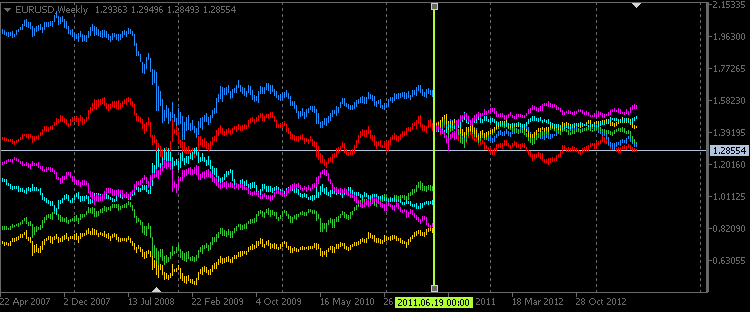Fig. 1 - Weekly timeframe in the "Vertical line" mode

On the screenshot below you can see the M30 timeframe in the Day mode, but this time inversion is enabled for symbols with USD as a base currency. In our case these are USDCAD (light blue candles) and USDCHF (purple candles).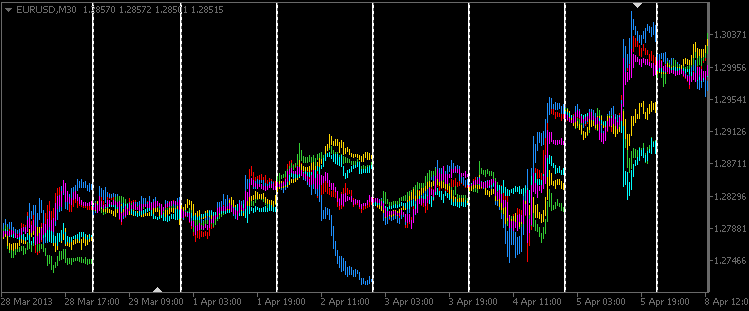Fig. 2 - M30 timeframe in the "Day" mode

### Conclusion

I think we have created a pretty interesting and informative tool for multi-currency analysis of price divergence. This indicator can be infinitely improved.

Translated from Russian by MetaQuotes Software Corp.
Original article: https://www.mql5.com/ru/articles/754

Attached files |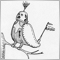Tales of Trading Robots: Is Less More?

Two years ago in "The Last Crusade" we reviewed quite an interesting yet currently not widely used method for displaying market information - point and figure charts. Now I suggest you try to write a trading robot based on the patterns detected on the point and figure chart.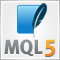SQL and MQL5: Working with SQLite Database

This article is intended for developers who would be interested in using SQL in their projects. It explains the functionality and advantages of SQLite. The article does not require special knowledge of SQLite functions, yet minimum understanding of SQL would be beneficial.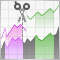Continuous futures contracts in MetaTrader 5

A short life span of futures contracts complicates their technical analysis. It is difficult to technically analyze short charts. For example, number of bars on the day chart of the UX-9.13 Ukrainian Stock index future is more than 100. Therefore, trader creates synthetic long futures contracts. This article explains how to splice futures contracts with different dates in the MetaTrader 5 terminal.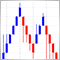Indicator for Renko charting

The article describes an example of Renko charting and its implementation in MQL5 as an indicator. Modifications of this indicator distinguish it from a classic chart. It can be constructed both in the indicator window and on the main chart. Moreover, there is the ZigZag indicator. You can find a few examples of the chart implementation.